• 当索赔次数数据存在过离散(over-dispersion)特征时,通常会采用负二项回归模型,但当索赔数据中同时又出现零膨胀(zero-inflated)问题时,负二项回归模型不再适合对这样的数据进行分析.在传统的零膨胀负二项回归模型为...
• 近期，需要使用零膨胀负二项回归模型。因此，找到R语言中的一个包：pscl。 首先，使用Rstudio下载此包。 install.packages("pscl") 该软件发表于下面的期刊。 Zeileis A, Kleiber C, Jackman S. Regression models ...
简介
近期，需要使用零膨胀负二项回归模型。因此，找到R语言中的一个包：pscl。
首先，使用Rstudio下载此包。
install.packages("pscl")

该软件发表于下面的期刊。
Zeileis A, Kleiber C, Jackman S. Regression models for count data in R[J]. Journal of statistical software, 2008, 27(8): 1-25.
详细使用可以参考：https://cran.r-project.org/web/packages/pscl/vignettes/countreg.pdf
案例
下面讲解一个自带的案例。
library(pscl)
data("bioChemists", package = "pscl")

fm_zinb <- zeroinfl(art ~ . | ., data = bioChemists, dist = "negbin")

在官方文档中提供了以下的一些方法用于查看模型的系数估计结果，预测，检验，AIC和BIC等。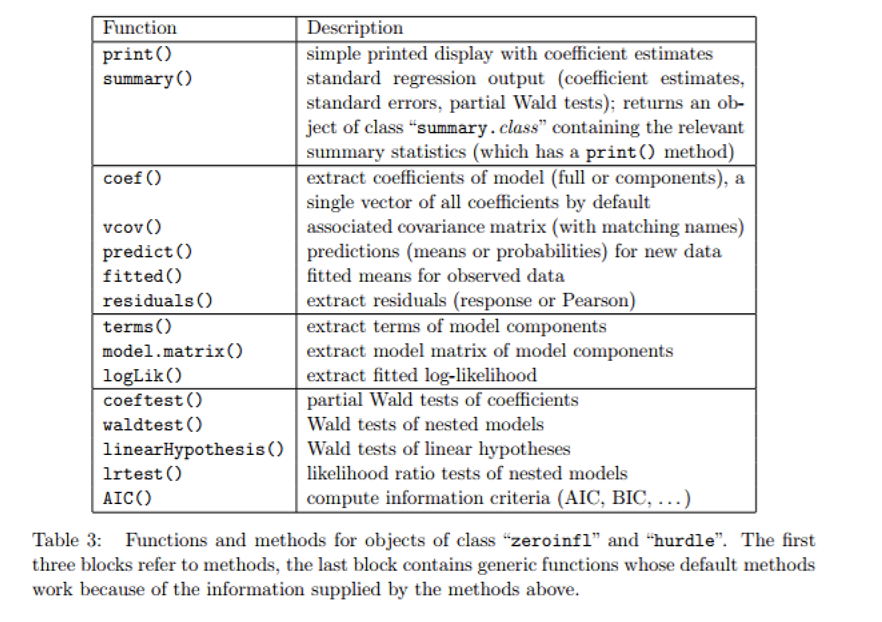首先，我们summary一下：
summary(fm_zinb)

可以看到如下结果：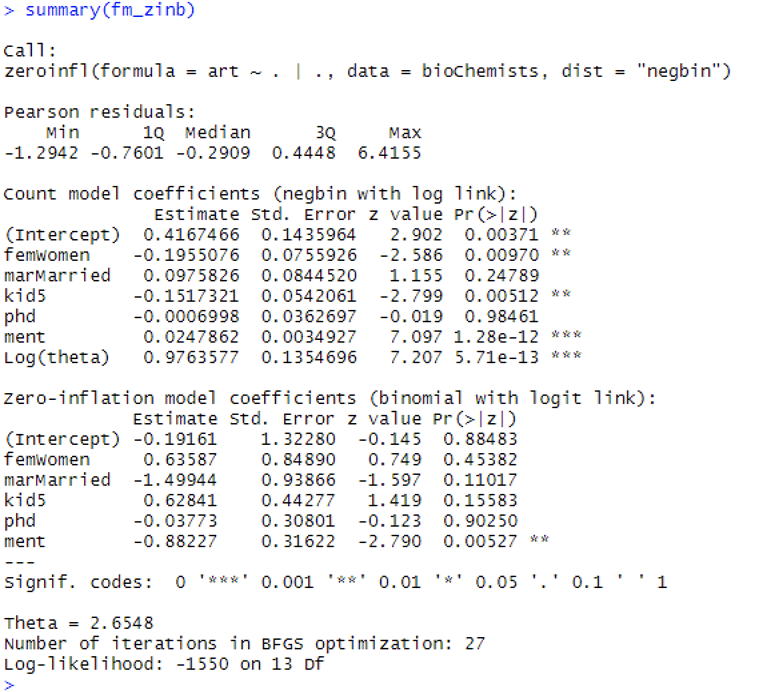其次，我想看看AIC和BIC的结果。
AIC(fm_zinb)
BIC(fm_zinb)

运行结果为：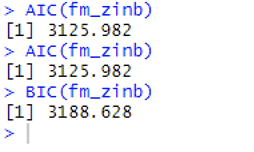展开全文R语言
• 负二项回归属于广义线性回归（GLM）的分支，与Logistic回归、Poisson回归等都属于计数数据模型的范畴，主要用于以分类变量、定序变量为因变量的回归分析之中。 负二项回归家族庞大，逐渐应用于社会科学领域各个学科...
• 'EMzeropoisson_mat.m'：零膨胀泊松回归。 'ZIPFA.m'：零膨胀泊松因子分析。 'cv_ZIPFA.m'：ZIPFA 上的交叉验证。 “示例代码.m”：“EMzeropoisson_mat.m”、“ZIPFA.m”、“cv_ZIPFA.m”的示例运行。matlab
• 零膨胀现象：在计数数据中，若0的个数明显多于泊松、负二项等标准离散分布随机产生的个数，称此现象为过多现象（zero-inflated, ZI） 零膨胀模型：伯努利分布与普通计数分布（泊松等）的混合分布，分为数据部分...
概念
零膨胀现象：在计数数据中，若0的个数明显多于泊松、负二项等标准离散分布随机产生的个数，称此现象为零过多现象（zero-inflated, ZI）零膨胀模型：伯努利分布与普通计数分布（泊松等）的混合分布，分为零数据部分及非零计数部分。结构零&抽样零：零膨胀模型零数据部分一部分来自于普通计数分布产生的抽样零，另一部分来自于额外得到的结构零。实际上，结构零可以看成由取值为零的退化总体产生，抽样零则是由非退化总体（Poisson分布等）产生。
例如：在调查一种药物的吸食状况数据时，且该药物吸食数据已经通过Poisson分布检验，则可以认为，抽样零表示Poisson分布中产生的零数据，结构零表示在调研过程中吸食过该类药物却表示从未吸食过的受访者所产生的额外零数据。
常用零膨胀回归模型
零膨胀泊松回归模型
Lambert（1992）描述了零膨胀泊松（ZIP）混合分布，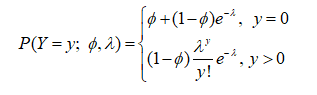记为Y~ZIP(Ф，λ) 当Ф=0时，ZIP分布退化为标准的Poisson分布；当0<Ф<1时，Ф越大，说明数据中的零膨胀现象越明显。 ZIP 数学期望与方差分别为：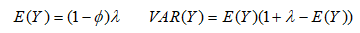在ZIP模型中，方差大于期望，这是由于模型中额外产生的结构零所致，从而可以解决事件发生数次零聚集所导致的过度离散现象。 为了讨论ZI数据中的因变量与自变量之间的关系，Lambert在ZI参数部分和泊松参数部分分别引入协变量，从而得到ZIP回归模型。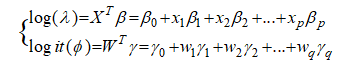其中，X为非零计数部分协变量，W为零数据部分协变量，β为非零计数部分回归系数，γ为零数据部分回归系数。 R语言实现：
library(pscl)

az1<-zeroinfl(Y~X1+X2+X3+X4+X5+X6+X7+X8+X9+X10+X11+X12)

summary(az1)
运行结果会出现两个模块，“Count model”表示非零计数部分，“Zero-inflation model”表示零数据部分。
零膨胀负二项回归模型
将ZIP中涉及的Poisson分布改为负二项分布形式，即可得到零膨胀负二项分布模型，记为ZINB，其相应的混合分布为：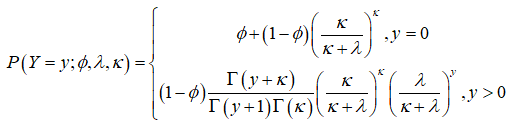其中，

δ

=

1

/

κ

δ=1/κ

为散度参数。 当散度参数

δ

→

0

δ→0

时，ZINB模型退化为ZIP模型。于是ZINB混合分布的回归模型与ZIP回归模型一致，即为：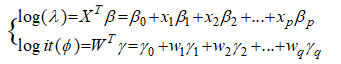R语言实现代码：
library(pscl)
az2<-zeroinfl(Y~X1+X2+X3+X4+X5+X6+X7+X8+X9+X10+X11+X12,dist
= "negbin")
summary(az2)
实例分析
Y表示某类药物吸食状况数据，协变量有年龄、性别等12个指标。用ZIP进行模型拟合。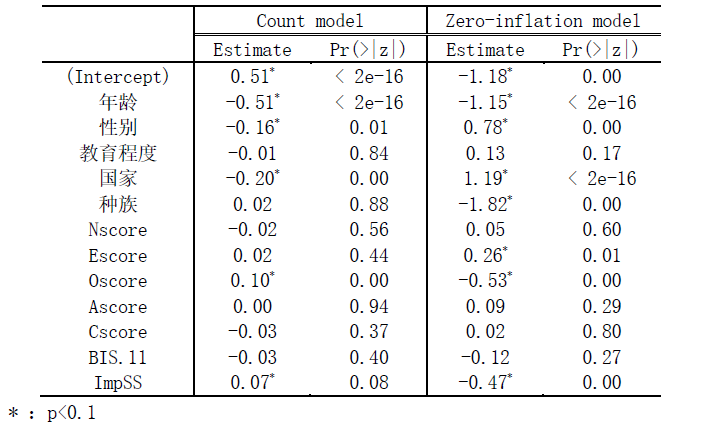模型结果为：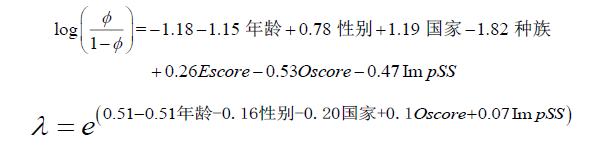在零数据部分，Ф表示的是零膨胀结构比例，在未吸食过该类药物的人群中，Ф越大，说明吸食该类药物的可能性越高。在非零计数部分，在已经吸食过该类药物的人群中，λ表示该类药物吸食频率均值，λ越大，说明吸食频率越高。
参考文献
解锋昌，韦博成，林金官.零过多数据的统计分析及其应用[M].科学出版社，2013吴喜之.复杂数据统计方法–基于R的应用[M].中国人民大学出版社,2015
展开全文R语言 统计学
• ????... 作者：郑浩文（中山大学）邮箱：... 1.2 零膨胀问题与 ZIP 1.3 模拟零膨胀数据 2. Stata 实操文章翻译 2.1 翻译说明 2.2 正文 2.3 译注及扩展 3. 参考文献 4. 相关推文 ????全文阅读：https://ww
🍎全文阅读：https://www.lianxh.cn/news/2144066aaa0b4.html

作者：郑浩文（中山大学）邮箱： zhenghw25@mail2.sysu.edu.cn

目录
1. 引入：计数模型与 ZIP
1.1 计数模型的使用1.2 零膨胀问题与 ZIP1.3 模拟零膨胀数据2. Stata 实操文章翻译
2.1 翻译说明2.2 正文2.3 译注及扩展3. 参考文献4. 相关推文
🍎全文阅读：https://www.lianxh.cn/news/2144066aaa0b4.html
展开全文stata 连享会
• 对Crash Frequency.xls文件的数据进行建模分析，并回答以下问题：1.1、首先导入相关数据1.2、描述性统计1.3、计算变量的方差膨胀因子，检查多重共线性问题1.4、采用负二项回归建模1.5、拟合泊松回归模型2、 ...


目录
1、 请采用计数数据分析模型（Count Data Model），对Crash Frequency.xls文件的数据进行建模分析，并回答以下问题：1.1、首先导入相关数据1.2、描述性统计1.3、计算变量的方差膨胀因子，检查多重共线性问题1.4、采用负二项回归建模1.5、拟合泊松回归模型
2、 Red light running.xls文件是研究人员对四个交叉口开展闯红灯调查的记录数据2.1、首先导入相关数据2.2、生成交叉口和年龄的哑变量2.3、描述性统计2.4、检查共线性2.5、建立二项logistics模型
3、 请基于Severity.csv文件，针对事故严重程度（severity变量）构建决策树模型：3.1、首先导入相关数据3.2、描述性统计3.3、开始构建决策树3.4、利用网格搜索，标定决策树最优超参数3.5、获得最优超参数：split criterion为gini，最大深度为9，最小划分样本数量为23.6、构建决策树3.7、画出决策树结构图3.8、解读决策树分类规则
4、 请基于Severity.csv文件，构建随机森林模型对事故严重程度的影响因素进行重要度排序：4.1、根据网格搜索选择最优参数4.2、得到最优模型参数4.3、计算impurity-based特征重要度和permutation特征重要度4.4、特征变量重要度排序结果
参考：

1、 请采用计数数据分析模型（Count Data Model），对Crash Frequency.xls文件的数据进行建模分析，并回答以下问题：
所采用计数数据分析模型的类型及原因；事故发生频率显著相关的因素；模型整体拟合优度的评价。
import pandas as pd
import numpy as np
import seaborn as sns
import matplotlib.pyplot as plt
import statsmodels.api as sm
from statsmodels.stats.outliers_influence import variance_inflation_factor
import warnings
warnings.simplefilter("ignore")
sns.set_style('whitegrid')

1.1、首先导入相关数据
df_1 = pd.read_excel('Crash frequency.xls')

1.2、描述性统计
可以看到，0值在Crash_Freq中的分布大于50%，且均值（1.096）远小于方差（3.802），可以考虑建立零膨胀模型。
def summary_statistics(df):
df_summary = df.describe().T
df_summary['std'] = df.var()
df_summary.columns = ['count', 'mean', 'var', 'min', '25%', '50%', '75%', 'max']
return df_summary

summary_statistics(df_1)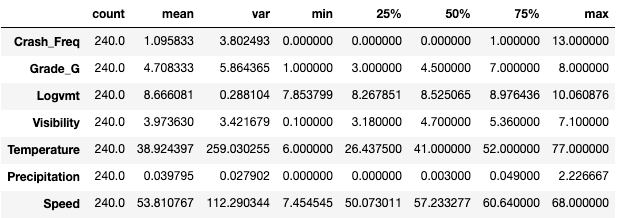1.3、计算变量的方差膨胀因子，检查多重共线性问题
可以看到，所有变量的方差膨胀因子（VIF）均小于5，说明没有多重共线性问题存在。
def cal_vif(df,col):
col.append('const')
df_vif = df.loc[:,col]
return pd.DataFrame({'variable':col,'VIF':[variance_inflation_factor(np.matrix(df_vif),i) for i in range(len(col))]}).iloc[:-1]

cal_vif(df_1,['Grade_G','Logvmt', 'Visibility', 'Temperature',
'Precipitation', 'Speed'])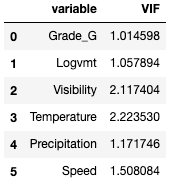plt.figure(figsize=(10,6))
sns.histplot(x='Crash_Freq',data=df_1,binwidth=0.5,color='blue')
plt.xticks(range(0,df_1['Crash_Freq'].max()+1),fontsize=15)
plt.yticks(fontsize=15)
plt.xlabel('Crash_Freq',fontsize=16)
plt.ylabel('Count',fontsize=16)
plt.show()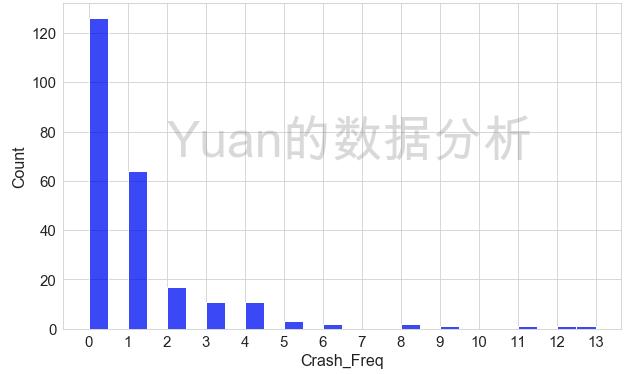1.4、采用负二项回归建模
model_1_nb = sm.NegativeBinomial.from_formula('Crash_Freq ~ Grade_G + Logvmt + Visibility + Temperature + \
Precipitation + Speed ',data=df_1).fit(method='ncg',maxiter=1000)
print(model_1_nb.summary2())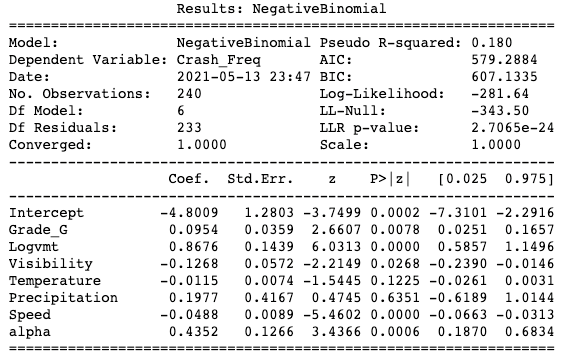模型结果表明:
事故发生频率与交通周转量及路段坡度显著正相关（即交通周转量越大、坡度越大的路段，事故发生的频率越高）;事故发生频率与平均能见度、平均气温以及平均速度显著负相关 （即平均能见度越低、气温越低以及平均速度越低的路段，事故发生率越高）。
模型拟合优度方面:
离散系数alpha在0.1%水平下显著，表明有必要采用负二项回归;模型的AIC与BIC分别为579.29和607.13，伪R方为0.180。
1.5、拟合泊松回归模型
为了更好地评价负二项回归模型的拟合优度，我们利用相同的变量拟合泊松回归模型
model_1_poisson = sm.Poisson.from_formula('Crash_Freq ~ Grade_G + Logvmt + Visibility + Temperature + \
Precipitation + Speed ',data=df_1).fit(method='ncg',maxiter=1000)
print(model_1_poisson.summary2())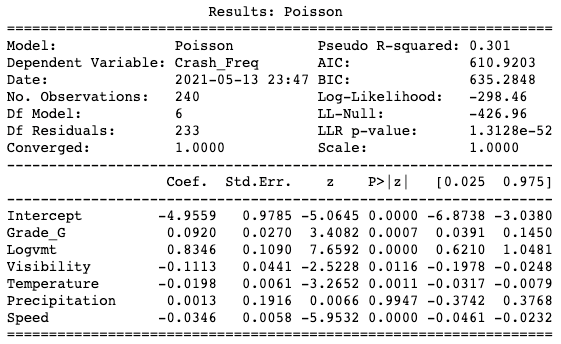可以发现泊松回归的AIC与BIC都明显大于负二项回归的AIC与BIC，再次表明负二项回归的拟合优度更高。
2、 Red light running.xls文件是研究人员对四个交叉口开展闯红灯调查的记录数据
其中，Running为二进制变量，表明观测对象是否闯红灯（1-闯红灯，0-未闯红灯）；Intersection为分类变量，表明交叉口编号；Local为二进制变量，表明观测对象是否是沪牌车（1-沪牌车，0-外地牌照车）；Passenger为二进制变量，表明观测对象是否载有乘客（1-载有乘客，0-未载有乘客）；Male为二进制变量，表明驾驶人是否为男性（1-男性，0-女性）；Age为分类变量，表明驾驶人的年龄分组。请基于此数据，采用恰当的分析模型，回答以下问题：
闯红灯行为显著相关的变量；4个交叉口间闯红灯行为是否有显著差异；计算在交叉口1，一位年龄为45岁的男性沪牌车驾驶员（未载客）其闯红灯的概率（给出计算公式）。
2.1、首先导入相关数据
df_2 = pd.read_excel('Red light running.xls')

2.2、生成交叉口和年龄的哑变量
def get_dummy_var(df,col):
dummy = pd.get_dummies(df[col])
new_col = []
for i in dummy.columns:
new_col.append(col + '_' + str(i))
dummy.columns = new_col
return dummy

df_2 = pd.concat([df_2,get_dummy_var(df_2,'Intersection'),get_dummy_var(df_2,'Age')],axis=1)

2.3、描述性统计
可以看到，三号交叉口和40岁年龄的比例最高，因此在建模时讲其设置为参照项。
summary_statistics(df_2)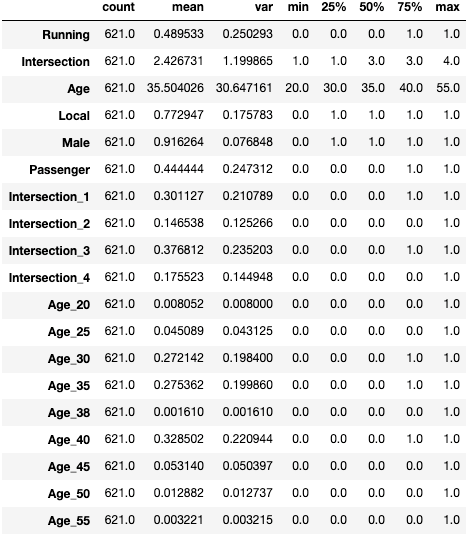2.4、检查共线性
所有变量VIF均小于5，表明没有多重共线性存在。
cal_vif(df_2,['Local', 'Male', 'Passenger',
'Intersection_1', 'Intersection_2', 'Intersection_4',
'Age_20', 'Age_25', 'Age_30', 'Age_35', 'Age_38','Age_45',
'Age_50', 'Age_55'])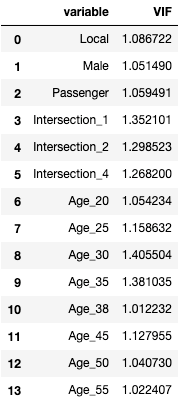2.5、建立二项logistics模型
model_2_logit = sm.Logit.from_formula('Running ~ Local + Male + Passenger + Intersection_1 + Intersection_2 + Intersection_4 + \
Age_20 + Age_25 + Age_30 + Age_35 + Age_38 + Age_45 + Age_50 + Age_55',data=df_2).fit(method='ncg',maxiter=1000)
print(model_2_logit.summary2())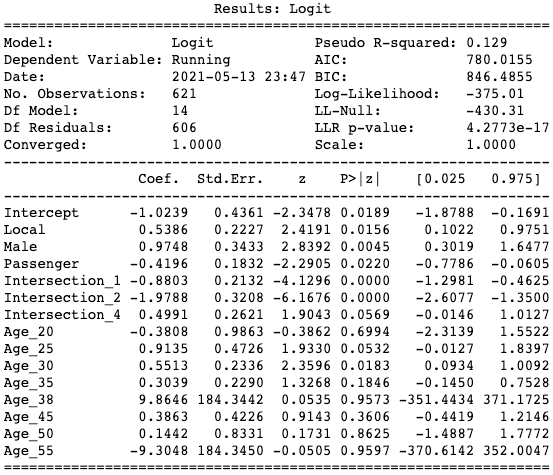通过模型结果可以发现，与闯红灯行为显著相关的变量有：
闯红灯行为与沪牌车、男性显著正相关，表明沪牌车与男性驾驶员更容易闯红灯;闯红灯行为与有载客显著负相关，表明有载客的车辆更不容易闯红灯;闯红灯行为与驾驶员年龄显著负相关，具体来讲，相比40岁的驾驶员，25和30岁的驾驶员更容易闯红灯，且25岁的驾驶员闯红灯的概率更高，而其余年龄的驾驶员在闯红灯行为方面与40岁的驾驶员没有显著区别。
4个交叉口间闯红灯行为是否有显著差异？回答：是
通过模型结果我们可以发现，Intersection_1, Intersection_2, Intersection_4三个变量均显著，这表明1，2，4号交叉口闯红灯行为与3号交叉口相比均有显著差异，进一步绘制这三个变量的Odds Ratio：

OR值（odds ratio）又称比值比、优势比，主要指病例组中暴露人数与非暴露人数的比值除以对照组中暴露人数与非暴露人数的比值，是流行病学研究中病例对照研究中的一个常用指标。

参考：https://www.douban.com/note/352258282/

举例： 共有10个male，10个female考大学， 被录取的结果为：7个male， 3个female 使用上面的结论： 从录取这个角度而言，而言odds(male) = 0.7/0.3 = 2.33 odds(female) = 0.3/0.7 = 0.428 所以录取的odds ratio为：OR = odds(male)/odds(female) = 2.37/0.42=5.44; 结论和意义：对一个male而言，录取的成功率比femal 高5.44倍。

from math import e,log

plt.scatter([-0.8803,-1.9788,0,0.4991],[4,3,2,1],color='k',marker='s')
plt.hlines(4,-1.2981,-0.4625,color='k',linewidth=1.5)
plt.hlines(3,-2.6077,-1.3500,color='k',linewidth=1.5)
plt.hlines(1,-0.0146,1.0127 ,color='k',linewidth=1.5)
for i in [log(0.1),log(0.5),log(1.5),log(2)]:
plt.vlines(i,0,5,linestyle='--',color='grey',linewidth=0.5)
plt.vlines(0,0,5,color='k',linewidth=0.5)
plt.ylim(0.5,4.5)
plt.grid(False)
plt.yticks(range(1,5),['Intersection_4', 'Intersection_3', 'Intersection_2', 'Intersection_1'],fontsize=14)
plt.xticks([log(0.1),log(0.5),log(1),log(1.5),log(2)],[0.1,0.5,1,1.5,2],fontsize=13)
plt.xlabel('Odds Ratio',fontsize=14)
plt.show()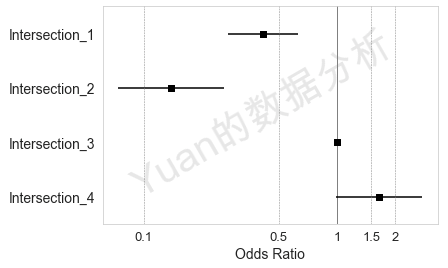可以发现，四个交叉口发生闯红灯行为的概率大小为交叉口4>交叉口3>交叉口1>交叉口2。
（3）计算在交叉口1，一位年龄为45岁的男性沪牌车驾驶员（未载客）其闯红灯的概率（给出计算公式）。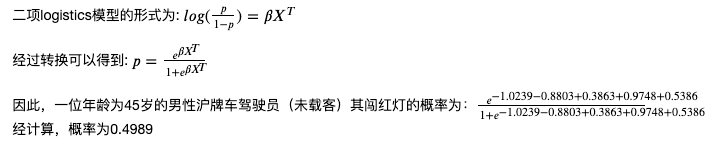3、 请基于Severity.csv文件，针对事故严重程度（severity变量）构建决策树模型：
给出Split Criterion计算依据;给出决策树结构图；对模型分类规则进行解释（解读三条规则即可）。
3.1、首先导入相关数据
df_4 = pd.read_csv('severity binary.csv')

### 变量编码
df_4['severity'] = df_4['severity'].map({'PDO':0,'INJ':1})
df_4['lane'] = df_4['lane'].map({'two':2,'thr':3})
df_4['snow_season'] = df_4['snow_season'].map({'dry':0,'snow':1})
df_4['ice'] = df_4['ice'].map({'no':0,'yes':1})
df_4['slush'] = df_4['slush'].map({'no':0,'yes':1})

3.2、描述性统计
可以看到，仅有7.3%的样本为受伤事故，在该场景下，我们希望尽可能预测出受伤事故，因此我采用召回率（Recall）作为模型性能的评价指标，以便更多的找到受伤事故样本。此外，对于所有样本，变量ice均为no，因此在建模过程中，我舍弃了该变量。
summary_statistics(df_4)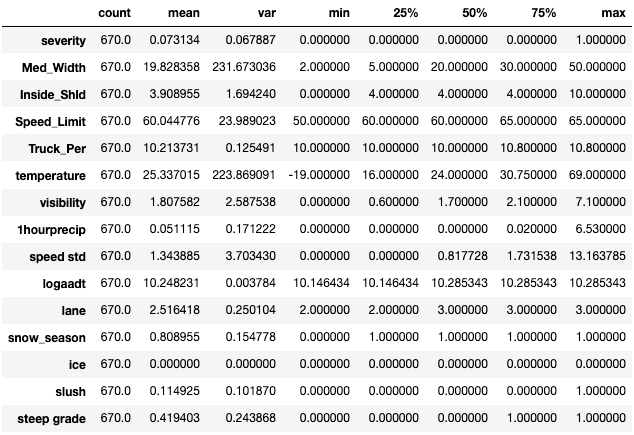3.3、开始构建决策树
from sklearn.tree import DecisionTreeClassifier,export_graphviz
from sklearn.model_selection import GridSearchCV,train_test_split
import graphviz

x = df_4.drop(columns=['severity','ice'])
y = df_4['severity']
name = x.columns

3.4、利用网格搜索，标定决策树最优超参数
cv_params = {'min_samples_split':range(1,20), 'max_depth':range(1,20),'criterion':['gini','entropy']}
ind_params = {'random_state': 10}
optimized_GBM = GridSearchCV(DecisionTreeClassifier(**ind_params),cv_params,scoring='recall', cv=5, n_jobs=-1, verbose=10)
optimized_GBM.fit(x, y)
print('最优分数:', optimized_GBM.best_score_)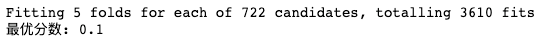3.5、获得最优超参数：split criterion为gini，最大深度为9，最小划分样本数量为2
print(optimized_GBM.best_params_)

结果：{'criterion': 'gini', 'max_depth': 9, 'min_samples_split': 2}
3.6、构建决策树
clf = DecisionTreeClassifier(criterion='gini', max_depth=9, min_samples_split=2,random_state=10)
clf.fit(x,y)

3.7、画出决策树结构图
dot_data = export_graphviz(clf, out_file=None, feature_names=name,
filled=True, rounded=True,  class_names=['PDO','INJ'],
special_characters=True)
graph = graphviz.Source(dot_data)
graph.render('决策树结构')
graph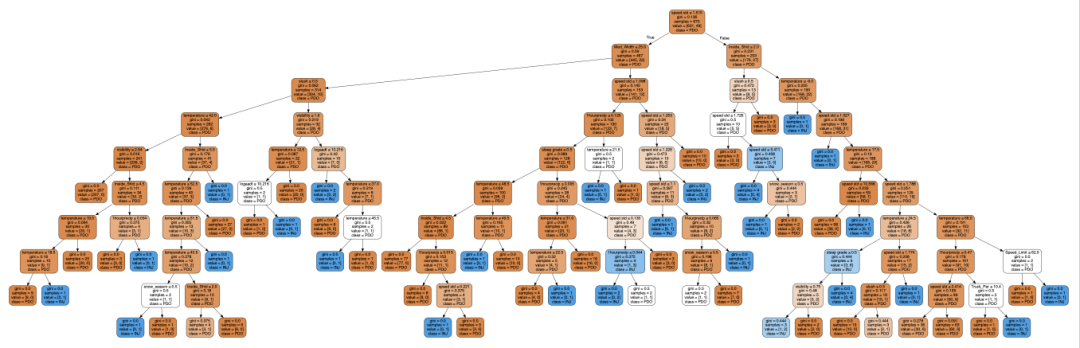3.8、解读决策树分类规则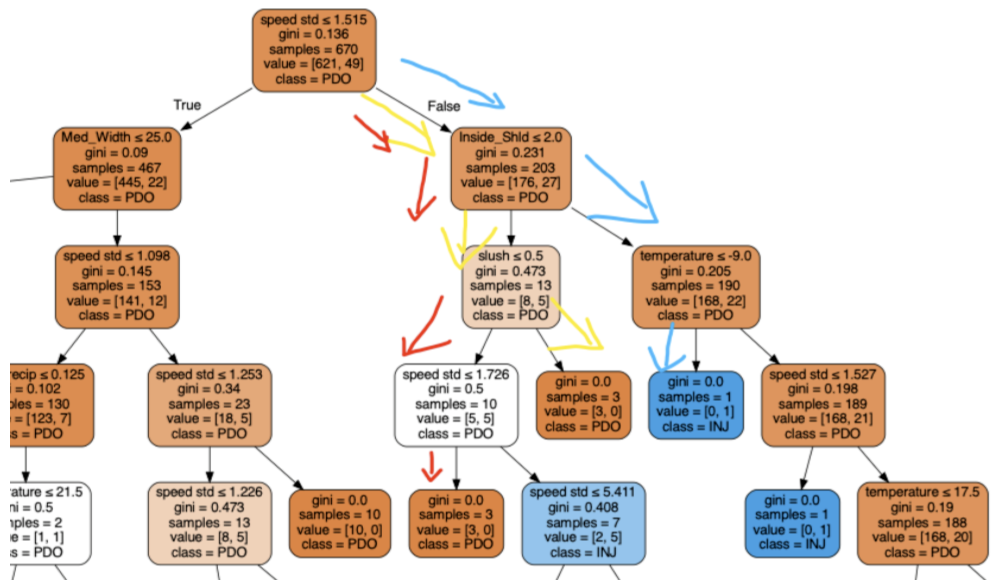对上述红、黄、蓝三个分类路径进行解读：
首先决策树根据运行速度方差进行样本划分，如果运行速度方差大于1.515，样本进入右侧分类路径，这同样符合常理，当道路运行速度方差大时，说明低速高速车辆同时存在，发生车祸时危险程度更大；对于右侧样本，继续根据路肩宽度进行划分，如果路肩宽度小于等于2，样本进入左侧划分区域，如果路肩宽度大于2，样本则进入右侧划分区域；对于速度方差大于1.515、路肩宽度小于等于2的样本，如果路面湿滑，则被预测为PDO样本，如果路面不湿滑且运行速度方差小于等于1.726，则被预测为PDO样本；对于速度方差大于1.515、路肩宽度大于2的样本，如果温度小于-9，则被预测为INJ样本。
4、 请基于Severity.csv文件，构建随机森林模型对事故严重程度的影响因素进行重要度排序：
给出随机森林设定参数；给出变量重要度排序结果。
4.1、根据网格搜索选择最优参数
通常，针对随机森林，有三个重要参数，分别为：弱学习器（决策树）数量，弱学习器（决策树）的深度（代表了弱学习器的复杂程度）以及每次进行节点划分时使用的特征数量。下面，我将利用袋外样本recall指标，对这三个参数的最优组合进行标定。其中，弱学习器数量设置为[100,1600,100]，最大特征数设置为[3,6,9]，弱学习器深度设置为[1,50,5]
from sklearn.metrics import recall_score,accuracy_score
from sklearn.ensemble import RandomForestClassifier
from sklearn.inspection import permutation_importance

gs = pd.MultiIndex.from_product([np.arange(100,1600,100),[3,6,9],np.arange(1,50,5)],names=['n_estimators','max_features','max_depth']).to_frame().reset_index(drop=True)

gs['oob_score'] = 0

### 开始网格搜索

from tqdm import tqdm

for i in tqdm(gs.index):
rf = RandomForestClassifier(n_estimators=gs.loc[i,'n_estimators'],max_features=gs.loc[i,'max_features'],max_depth=gs.loc[i,'max_depth'],oob_score=True,random_state=20,n_jobs=-1).fit(x,y.ravel())
gs.loc[i,'oob_score'] = recall_score(y,[0 if i >= 0.5 else 1 for i in rf.oob_decision_function_ ])

plt.figure(figsize=(10,6))
plt.scatter(100,gs[gs['max_depth']==16]['oob_score'].max(),color='red',label='Optimal point',zorder=1)
plt.legend()
sns.lineplot(x='n_estimators',y='oob_score',data=gs[gs['max_depth']==16],hue='max_features',palette=['C0','C1','C2'],zorder=0)
plt.xticks(range(100,1600,100),fontsize=12,rotation=45)
plt.yticks(fontsize=12)
plt.xlabel('n_estimators',fontsize=15)
plt.ylabel('oob_score',fontsize=15)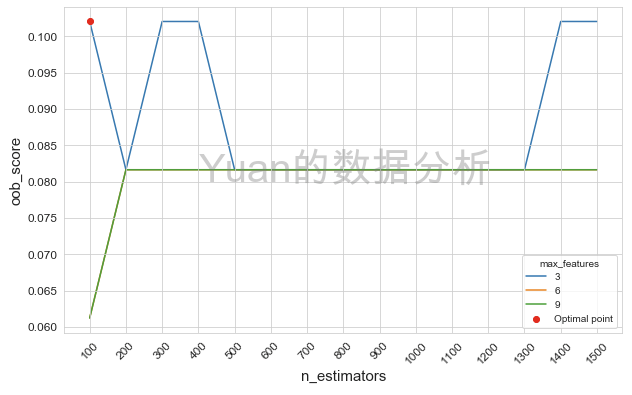4.2、得到最优模型参数
100个弱学习器，最大特征数为3，决策树深度为16
rf = RandomForestClassifier(n_estimators=100,max_depth=16,max_features=3,random_state=20)
rf.fit(x,y)

4.3、计算impurity-based特征重要度和permutation特征重要度
其中，impurity-based特征重要度根据每个节点根据特征划分后获得的基尼增益计算；permutation特征重要度为将特征随机打乱，计算打乱后模型性能的变化。
feature = pd.DataFrame(list(zip(name,rf.feature_importances_)),columns=['Variable','Relative Importance (impurity-based)']).sort_values(by='Relative Importance (impurity-based)')
perm_imp = permutation_importance(rf,x,y,random_state=10,n_repeats=99)
feature_perm = pd.DataFrame(list(zip(name,perm_imp['importances_mean'])),columns=['Variable','Relative Importance']).sort_values(by='Relative Importance')
feature_perm['Relative Importance'] = feature_perm['Relative Importance']/feature_perm['Relative Importance'].sum()
feature_perm.columns = ['Variable', 'Relative Importance (permutation)']
feature = pd.merge(feature,feature_perm,on='Variable',how='left')

plt.figure(figsize=(6,8))
plt.barh(np.arange(13)+0.15,feature['Relative Importance (impurity-based)'],height=0.3,color='k')
plt.barh(np.arange(13)-0.15,feature['Relative Importance (permutation)'],height=0.3,color='C3')
plt.ylim(-0.5,12.5)
plt.yticks(range(13),feature['Variable'],fontsize=13)
plt.xticks(fontsize=14)
plt.xlabel('Relative Importance',fontsize=16)
plt.yticks(fontsize=16)
plt.legend(['impurity-based','permutation'],fontsize=13)
plt.show()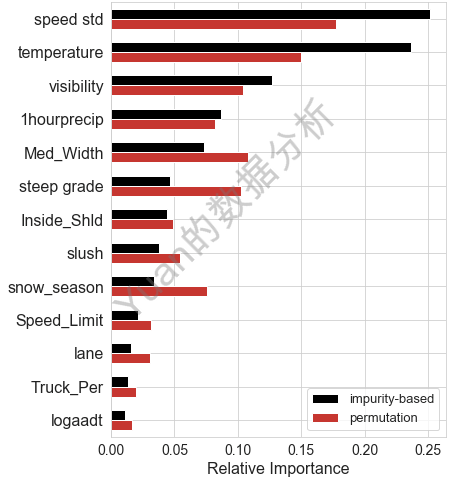4.4、特征变量重要度排序结果
可以看出，总体上无论是impurity-based还是permutation方法计算的特征重要度，速度的标准差和温度都是最重要的两个变量，其次是能见度、降雨量、中央隔离带宽度和是否陡坡；在两种方式计算的特征重要度中，限速、车道数、货车比例和日均流量对数均为四个特征重要度最小的变量。
参考：
https://mp.weixin.qq.com/s/3DHEAumY0F0K31Pb1TjruQ
展开全文机器学习 决策树 随机森林
• 利用R语言对贷款客户作风险评估（下）——零膨胀回归分析 前言 上一篇的分类预测是决定好坏客户的初步判断, 不足以直接决策, 因此还需要进一步分析. 通过随机森林, 对影响好坏客户的解释变量的重要性进行排序. 由...r语言
• 选取初始释放瓦斯膨胀能作为因变量,并从近几年来的初始释放瓦斯膨胀能的实测...且建立了预测模型,并对预测结果进行分析验证,预测的精度可以满足工程的需要,说明利用逐步回归分析对初始释放瓦斯膨胀能进行预测是可行的。
• 原文链接：... 泊松模型 proc fmm data = tmp1 tech = trureg; model majordrg = age acadmos minordrg logspend / dist = truncpoisson; probmodel age acadmos minordrg logspend;...
• 广义线性模型（GLM）是通过连接函数,把自变量线性组合和因变量的概率分布连起来,该概率分布可以是高斯分布、二项分布、多项式分布、泊松分布、伽马分布、指数分布。连接函数有：R语言
• 回归分析结果表明：膨胀力、膨胀回归曲线线性相关显著，膨胀力与干密度的关系为正相关，而膨胀力与含水量及膨胀量与压力的关系为相关．对于同一种膨胀黏土，含水量和干密度的大小是影响膨胀力、膨胀量大小的主要...
• （1）Estimates：SPSS默认输出，输出与回归系数相关的统计量： 回归系数（偏回归系数）、回归系数标准误差、标准化回归系数、回归系数显著性检验的t统计量和概率p值，各解释变量的容忍度。（2）Co...
• 原文链接：http://tecdat.cn/?p=6145... 泊松模型 proc fmm data = tmp1 tech = trureg; model majordrg = age acadmos minordrg logspend / dist = truncpoisson; probmodel age acadmos minord...
• 【数据分析】从开始带你了解商业数据分析模型——线性回归模型 1 摘要 随着数据导向型决策、数据科学、大数据分析等话题日益火热，各行各业都开始关注数据分析这个课题。 **数字化转型成了很多企业在未来十年的...
• 1. 如何在Altair KnowledgeStudio平台应用线性回归 1.1. 为什么选择Altair KnowledgeStudio平台 绝大多数的数据分析项目都是遵照着CRISP-DM的行业标准流程 （详情可见：https://zhuanlan.zhihu.com/p/107757240）。 ...大数据 经验分享
• 假设检验：模型显著性检验——F检验（利用statsmodels中建立模型的summary/summary2方法）偏回归系数显著性检验——t检验（利用statsmodels中建立模型的summary/summary2方法）诊断：正态性检验：方法实现PP图/QQ图...
• 文章目录线性回归、岭回归和LASSO回归模型1 线性回归模型1.1 一元线性回归模型1.1.1 模型介绍1.1.2 参数求解1.1.3 python实现1.2 多元线性回归模型1.2.1 模型介绍1.2.2 模型参数求解1.3 回归模型的假设检验1.3.1 ...机器学习 线性代数 python
• 关注一下~，更多商业数据分析案例等你来撩 构建多元线性回归模型时，如果能够充分的使用已有变量，或将其改造成另一种形式的可供使用的变量，将在一定程度上提高模型精度及其泛化能力。因为数据集中的名义变量(或叫...数据建模 数据挖掘 python
• 标准线性回归2.1 线性回归（二元一次函数，此时$w$是一个数）2.1.1均方误差最小化2.1.2最小二乘参数估计2.2 多元线性回归（此时$w$是一个向量）3 局部加权线性回归3.1 基本思想3.2 权重怎么取？3.3 [机器学习-核函数...
• 多重线性回归分析的四大纪律三注意 鉴于多重线性回归已经到了滥用的程度，特总结一下在使用线性回归时需要注意的问题，总结为四大纪律加三注意。 四大纪律 四大纪律之一： 自变量与因变量之间要存在线性关系...
• 回归模型的参数设置 1，在回归模型前设置类型，角色分配：1个目标，多个输入，无关的数据角色选择“无”； 2，在【字段】中选择使用预定角色； 3，在【模型】中按需求选择建模方法； 注：当模型不再将新字段纳入模型...
• 1. RNA-seq为什么是负二项分布？ 2.负二项分布解释 3.DESeq2基于负二项分布找差异基因 4.生物信息学基础知识1 5.生物信息学基础知识2 6.广义线性回归模型 7.广义线性回归模型 ......